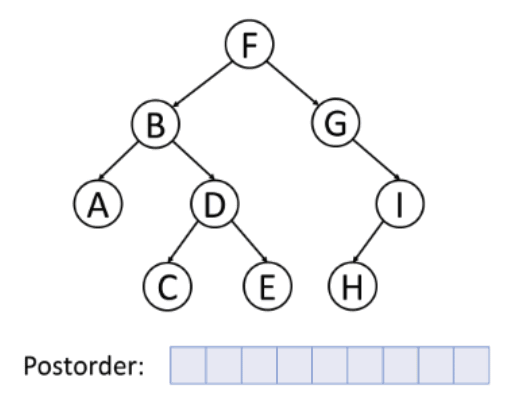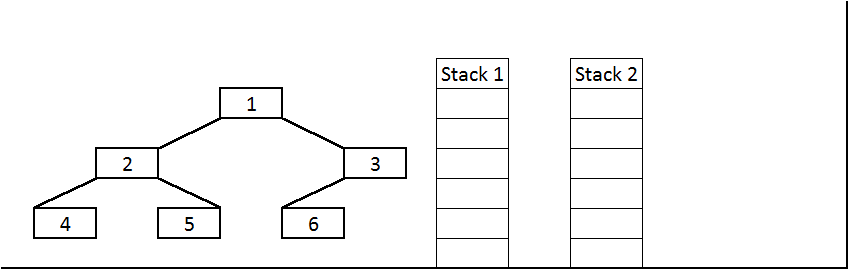# Iterative Postorder Traversal of Binary tree

## Introduction

Our today’s topic is Iterative Postorder Traversal of a Binary tree. If you are reading this article, you must be familiar with binary trees, which is the prerequisite for this topic. Let’s understand the difference of traversal between linear data structures and non-linear data structures. Unlike linear data structures (Array, Linked List, Queues, Stacks, etc.), which can only be traversed in one logical manner, trees can be traversed in various ways, which are:

Depth First Traversal: In depth-first search (DFS), the search tree is deepened as much as possible before going to the next sibling.

• Pre-order traversal ( Root Left Right )
• In-order traversal ( Left Root Right )
• Postorder traversal ( Left Right Root )

Breadth-First Traversal: In Breadth-first search(BFS), the nodes are traversed in a level-wise order.

Although there is a possibility to perform a post order traversal of the binary tree recursively, in this article we’ll be exploring post-order traversal using the Iterative approach, which will use stacks to perform the traversal of the tree.

Before any further due, let’s get started:

## What is a Postorder Traversal?

A binary tree postorder traversal is a method of listing all of the tree's leaves and nodes so that for each node, all of its left children come before all of its right children, which come before the node itself. In simple words, Postorder traversal is a binary tree traversal approach in which you visit the left subtree first, then the right subtree, and finally the root.

Consider the following example of a binary tree traversal utilising the postorder.Source:- wikimedia

In the preceding example, because the leftmost node is A, it is visited first. Following that, since D is the root node of C and E, the nodes C and E will be visited before the D node. The B node is now the last one to be visited in the left subtree. Now, the left subtree is visited, and because the algorithm expects the root to be visited last, it moves to the right subtree and processes each node in the same manner as it did for the left subtree.

Now that you are familiar with postorder traversal let’s look at the approaches we can perform to traverse a binary tree. As we are attempting to traverse the tree iteratively, thus we’ll be needing an auxiliary stack to process each node.

## Approaches

1. Using two stacks: In this section, we’ll learn how two-stacks can assist in traversing the binary tree.
2. Using one stack: This is the more efficient approach to solving this problem. We have discussed this approach in another blog.

### Method 1: Using two stacks

We can use two stacks to implement the Postorder traversal. The goal is to acquire the reversed postorder elements in the stack and then pop the elements one by one from the stack, as the stack adheres to the LIFO principle. The question of how to obtain the reversed order of the postorder items emerges. This problem's answer can be reached by combining two stacks. The second stack is utilised to obtain the postorder items in reverse order.

The steps for implementing postorder utilising two stacks are as follows:

1 - We'll start by pushing the root to the top of the stack.

2 - Second, we'll run a while loop that will keep running until the first stack becomes empty and process the below steps.

• A node from the first stack is popped and pushed into the second.
• Push the left and right child of the popped node to the first stack.

3 - The data from the second stack will be printed.Source:- Algorithms@tutorial Horizon

Consider the above example, and let’s try to understand each step in detail before moving to the Implementation:

1. Push 1 to the first stack.

First stack: 1

Second stack: Empty

2. Pop 1 from the first stack and push it to the second stack.

Push left and right children of 1 to the first stack.

First stack: 2, 3

Second stack: 1

3. Pop 3 from the first stack and push it to the second stack.

Push the left child of 3 to the first stack.

First stack: 2, 6

Second stack: 1, 3

4. Pop 6 from the first stack and push it to the second stack.

First stack: 2

Second stack: 1, 3, 6

6. Pop 2 from the first stack and push it to the second stack.

Push left and right children of 2 to the first stack.

First stack: 4, 5

Second stack: 1, 3, 6, 2

7. Pop 5 from the first stack and push it to the second stack.

First stack: 4

Second stack: 1, 3, 6, 2, 5

8. Pop 4 from the first stack and push it to the second stack.

First stack: Empty

Second stack: 1, 3, 6, 2, 5, 4

The algorithm stops here since there are no more items in the first stack.

Observe that the contents of the second stack are in postorder fashion. Print them.

### Implementation in C++

#### Output

Time Complexity:- The time complexity is O(n) (where n is the number of nodes in the tree) because of the work we do in the while loops.

Space Complexity:- The space complexity is O(n) (where n is the number of nodes in the tree) because of the space taken by the two stacks.

To know more about conceptual knowledge and the implementation of the code, watch this video tutorial.

### Implementation in Java

Output

Time Complexity:- The time complexity is O(n) (where n is the number of nodes in the tree) because of the work we do in the while loops.

Space Complexity:- The space complexity is O(n) (where n is the number of nodes in the tree) because of the space taken by the two stacks.

Now, you have understood the approach; it’s time to implement the code on your own in your favourite programming language on CodeStudio. Complete your turn until it’s too late.1. When to use postorder traversal?
If you know you need to inspect all the leaves before any nodes, you choose post-order, so you don't waste time inspecting roots in search of leaves.

2. What is meant by traversal?
Tree traversal is the process of visiting each node in a tree exactly once. If a search results in a visit to all the nodes, it is called a traversal.

3. What is recursive and non-recursive tree traversal?
Recursive traversals are easier to implement because you only have to worry about one node, and they use the stack to store the state for each call, making them faster.
However, non-recursive functions require you to store a list of all nodes for each level, and they can be far more complex than recursive ones.

4. How does post-order traversal work?
The root node is visited last in this traversal technique. This is accomplished by first traversing the left subtree, then the right subtree, and ultimately reaching the root node.

5. What are the three common types of traversals?

Inorder

1. left-subtree --> visit the root --> right-subtree

Preorder

2. visit the root --> left-subtree --> right-subtree

Postorder

3. left-subtree --> right-subtree --> visit the root

For example:-

• inorder: {5 , 15 , 18 , 20 , 25 , 30 , 35 , 40 , 45 , 50 , 60}
• preorder: {30 , 20 , 15 , 5 , 18 , 25 , 40 , 35 , 50 , 45 , 60}
• postorder: {5 , 18 , 15 , 25 , 20 , 35 , 45 , 60 , 50 , 40 , 30}

## Key takeaways

To summarize, we’ve covered the tree traversal iteratively using the postorder DFS based on LRR( Left right root) algorithm. The postfix expression of an expression tree can be obtained using postorder traversal. Moreover, postorder traversal is also used when there is a need to visit the child nodes before the Root node. Postorder traversal is complicated among all the other traversals but is efficiently utilized in computer science. Along with that, a tree can be deleted from leaf to Node by using the postorder traversal.

So, in this article, we looked at postorder traversal with two stacks; however, there is a more interesting and memory-friendly way to traverse the tree with a single stack.

Continue reading the fantastic articles to improve your programming expertise in all areas.

Enroll in our top-tier courses taught by the best faculty to unleash the potential of learning.

Happy Leaning Ninja!